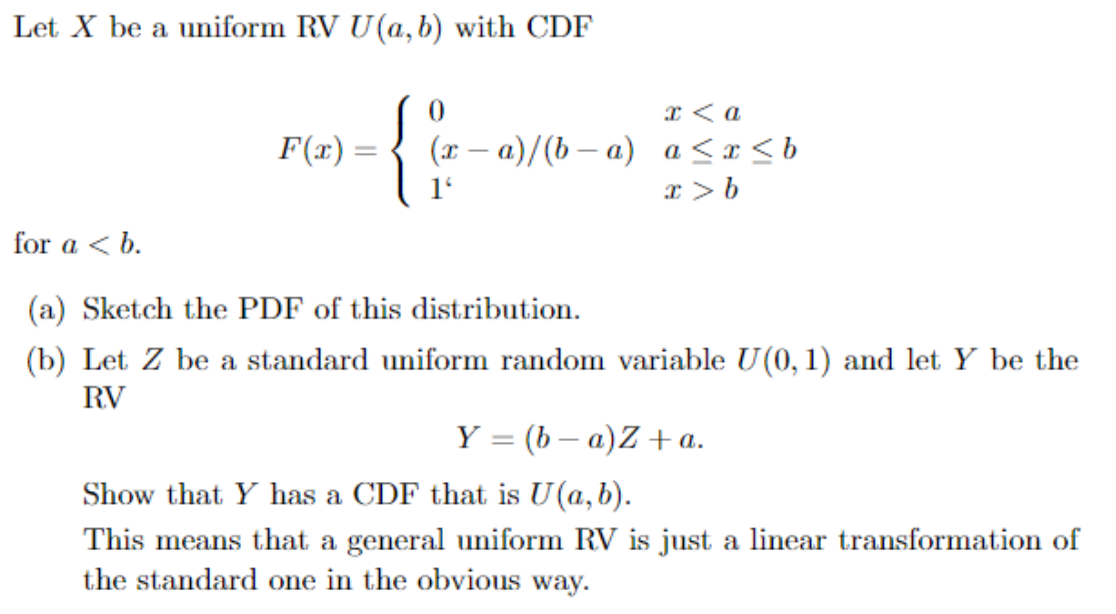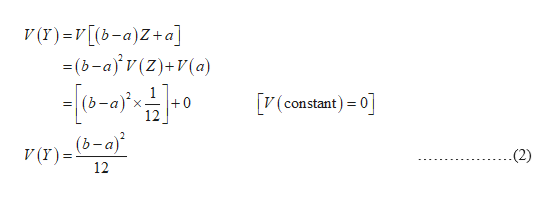# Let X be a uniform RV U(a, b) with CDF{0a(r-a)/(b a) a

Question

Show that Y has a CDF that is U(a,b).help_outlineImage TranscriptioncloseLet X be a uniform RV U(a, b) with CDF {0 a (r-a)/(b a) a
check_circleExpert Solution
Step 1

a.

Show the graph of the probability density function of Uniform distribution.

From the given information, the random variable X be a uniform random variable, U(a,b) and its probability density function is  f(x)=1/(b-a)

The graph of the probability density function is,

Step 2

b)

From the given information, Y=(b-a)Z+a and Z is a standard uniform random variable U(0,1).

The probability density function for Z is, f(Z)=1/(1-0)=1. The mean for Z is 1/2 and the variance for Z is 1/12.

The mean for Y=(b-a)Z+a is,

Step 3

The variance for Y=(b-a)Z+a is,

...help_outlineImage TranscriptioncloseV (r)=V[(b-a)Z+a] =(b-a)v(Z)+V(a) (constant) 0 (b-a)x +0 12 (b-a) V (r) = (2) 12 fullscreen

### Want to see the full answer?

See Solution

#### Want to see this answer and more?

Solutions are written by subject experts who are available 24/7. Questions are typically answered within 1 hour*

See Solution
*Response times may vary by subject and question
Tagged in

### Other